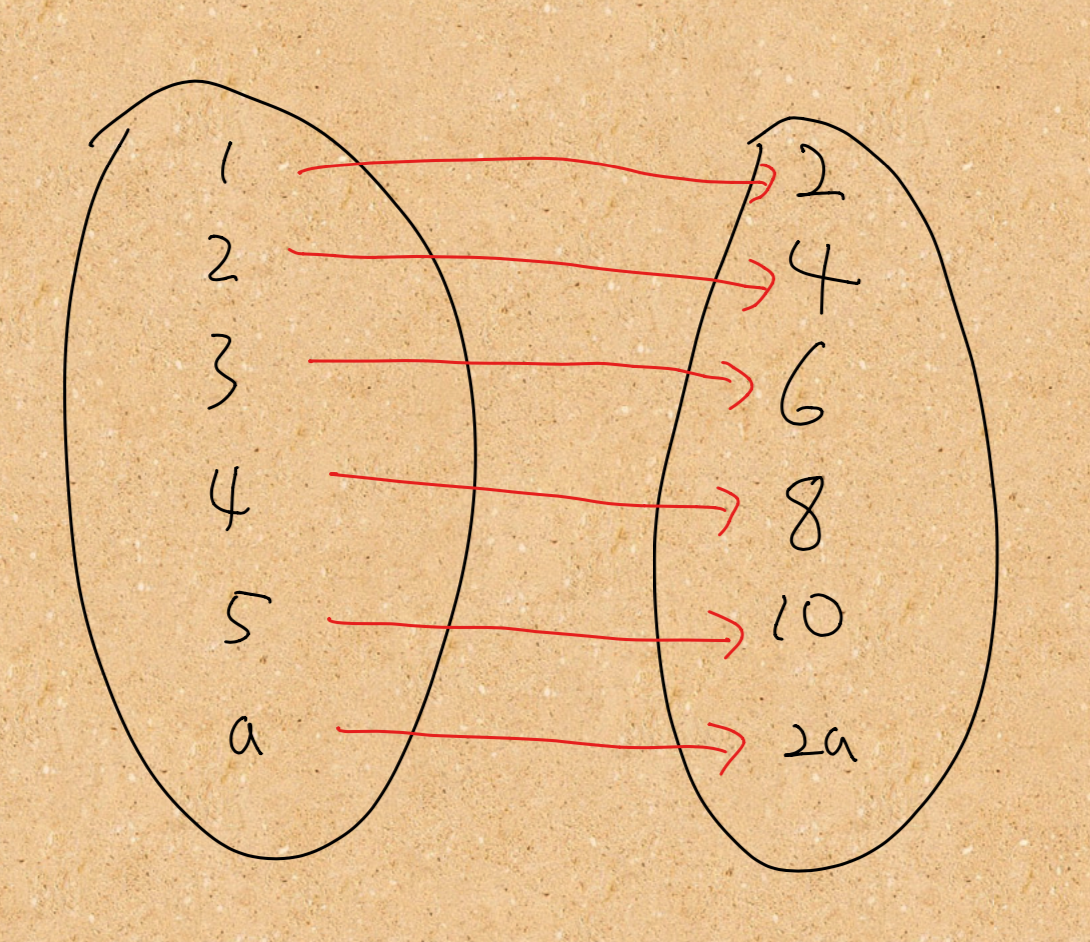#DAY 1
1
Software Development

# Intro

• Kotlin functional programming 基本語法
• 基本概念，包含 pure function、immutability 等等
• Category theory 入門
• 實戰運用

• Kotlin 程式語言基礎
• RxJava 原理與 Best practice

# Function type

Functional Programming 中的主角 - Function， 在 Kotlin 中是一個 first class citizen，這是什麼意思呢？對於我，甚至是大部分的人，第一個學習的語言應該會是“物件導向”的程式語言。這類的語言都有共同的特性：建立物件並將其指派到一個變數中，如下方程式碼所示

``````class Point(val x: Int, val y: Int)

fun main() {
val a: Point = Point(0, 0)
val b: Point = Point(0, 1)
val c: Int = 4
}
````````````val add: (Int) -> Int = fun(x) { x + 1 }
val minus: (Int) -> Int = {x -> x - 1 }               //lambda，通常會使用這形式
val printFun: (String) -> Unit = { x -> print(x)}     //也可以沒有回傳值，這時候會是 Unit
val helloWorld: (Unit) -> Unit = { print("Hello world")}   //輸入也可以是 Unit
val helloWorld2: () -> Unit = { print("Hello world")}      //當輸入是 Unit 時可以省略
val createPoint: (Int, Int) -> Point = { x, y -> Point(x, y)}   //多個輸入參數
val square: (Int) -> Int = { it*it }                          //如果只有一個參數的話可以簡化成 it
val square2 = { x: Int -> x*x }.                               //如果型別可以推斷的出來的話，function type 可以省略
``````

# Higher order function

``````// 輸入 logFun 是 Function Type
fun addOne(a: Int, logFun: (String) -> Unit): Int {
val result = a + 1
// 使用 invoke () 來執行 function
logFun.invoke(result.toString())
return result
}

// 回傳值是 Function Type
fun getCountDownListener(): (Int) -> Unit {
return { count: Int -> textView.text = count.toString }
}
``````

# Extension function

``````fun Canvas.withTranslation(
x: Float = 0.0f,
y: Float = 0.0f,
block: Canvas.() -> Unit
) {
val checkpoint = save()
translate(x, y)
try {
block()
} finally {
restoreToCount(checkpoint)
}
}

``````

``````fun ((Int) -> Int).double() = { a: Int -> this(a) * 2}

fun main() {
val addThree = { a: Int -> a + 3}
}

// 猜猜看會印出甚麼吧！
``````

# Infix function

``````fun main() {
}

// 請注意 infix function 一定同時會是 extension funcion
infix fun Int.add(another: Int) = this + another
``````

# Function reference

``````fun main() {
printEquation(4, ::divideTwo)
}

fun printEquation(input: Int, equation: (Int) -> Int) {
println(equation(input))
}

fun divideTwo(a: Int) = a / 2
``````

0